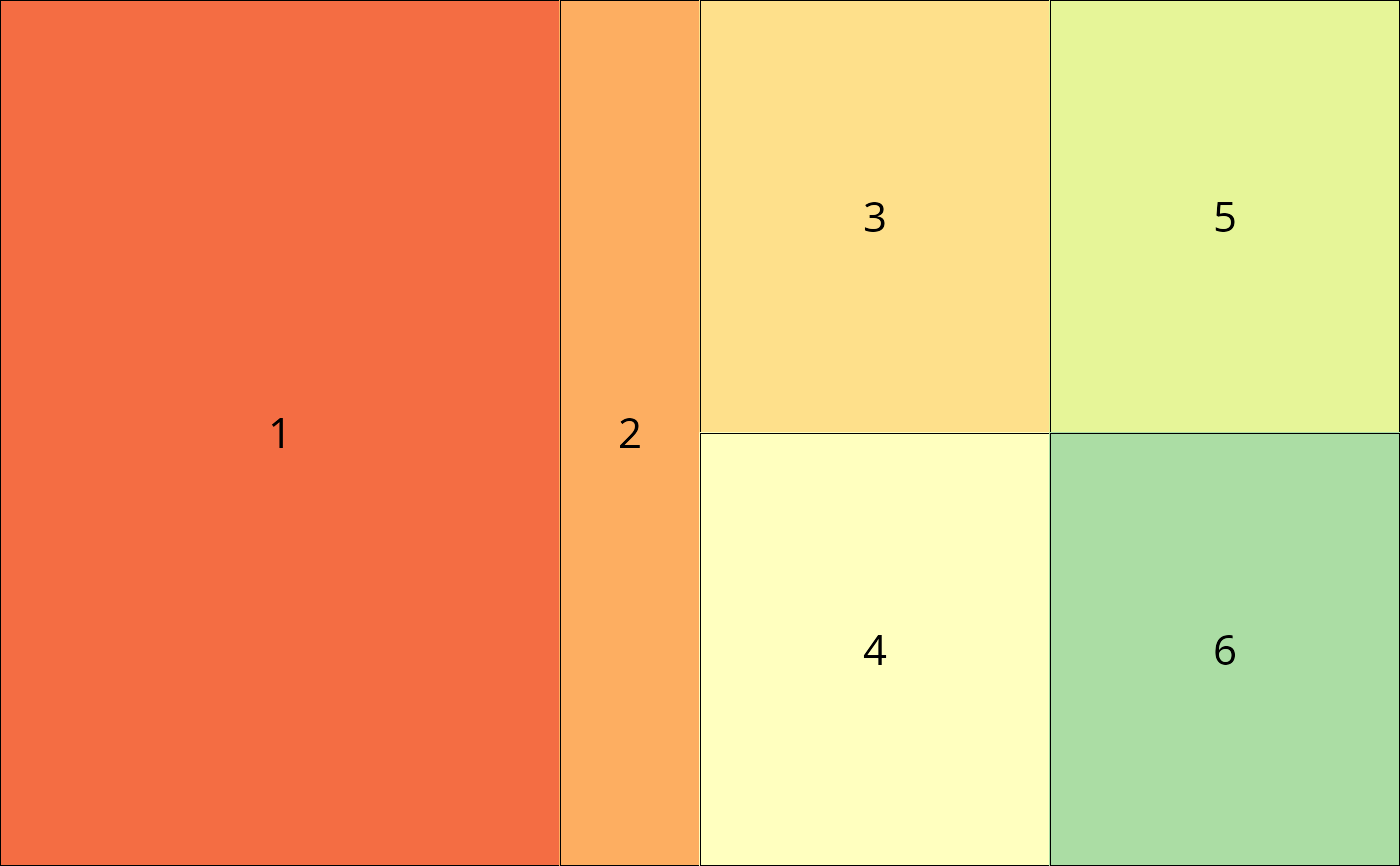Take two Layout objects and combine by rows.

lay_bind_col(x, y, widths = c(1, 1), addmax = TRUE)

layColBind(x, y, widths = c(1, 1), addmax = TRUE)

## Arguments

x object of class Layout object of class Layout a vector with relative widths used in combining the x and y layouts. if true (default) the ids of the plots in the second layout will be shifted by the number of plots in the first layout.

## Examples

l1 = lay_new(matrix(c(1:2),ncol = 2),widths=c(4,1))
l2 = lay_new(matrix(c(1:4),ncol = 2),widths=c(1,1))
lb = lay_bind_col(l1,l2)
lay_show(lb)